# Measurements In Electrical Engineering

## Powers of Ten

A numeral system is a method of using certain digits to represent numerical quantities. Currently, the dominant numeral systems are the decimal (or base-ten) system and the binary (or base-two) system. Nowadays people use the decimal system for almost everything; we also interact frequently, though usually indirectly, with the binary system, which is the basis of the computational activity performed by digital circuits.

As the name implies, the decimal system is built around the number ten, and engineers commonly use powers of ten when working with large numbers. A power of ten is expressed in writing as “10” along with a superscript number indicating how many tens should be multiplied together. Thus, 101 = 10, 102 = 10 × 10 = 100, and 103 = 10 × 10 × 10 = 1000.

The following large numbers are frequently expressed as powers of ten (or as prefixes, discussed later in this page, that correspond to a power of ten).

 Standard Notation Power of Ten Notation 1,000 (one thousand) 103 1,000,000 (one million) 106 1,000,000,000 (one billion) 109

Powers of ten can also be used for very small numbers. The concept is the same, but the superscript number has a negative sign that causes the overall expression to become the denominator of a fraction in which the numerator is 1. For example: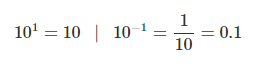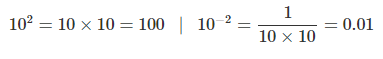The following small numbers are frequently expressed as powers of ten or as the corresponding prefixes:

 Standard Notation Power of Ten Notation 0.001 (one thousandth) 10-3 0.000001 (one millionth 10-6 0.000000001 (one billionth) 10-9

## Scientific Notation

We can represent any value by combining these powers of ten with other numbers. For example: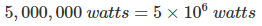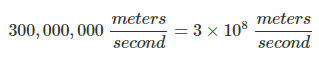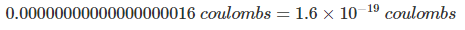This method of writing (and saying) numbers is called scientific notation. It is widely used in science and engineering, and (as you can see in the third example), it can save a lot of time and space when we’re working with numbers that are extremely small or extremely large.

Scientific notation also helps us to focus on the more important information. In some cases, the important information is the power of ten.

For example, if we’re discussing the electricity-generating capacity of a nuclear power plant, we might see the value 2.7 × 109 W. If we write this as 2,700,000,000 W (or worse, 2700000000 W, without the commas), we will be focusing on the “27.” We will readily notice a change from “27” to “28,” but we could easily fail to notice the loss of a zero. This is a problem, because losing one zero is a much more significant change than going from “27…” to “28….” If we use scientific notation, the loss of a zero is more apparent because it makes the expression change from 2.7 × 109 to 2.7 × 108.

There are also situations where we want to temporarily ignore the power of ten. Let’s say that we are adding the numbers 10000000, 18000000, and 23000000. This is actually an easy computation because the three numbers have the same power of ten, but before we can perform the addition we have to count all the zeros in each number (and then worry if perhaps we miscounted). If we use scientific notation, the numbers become 10 × 106, 18 × 106, and 23 × 106. Now we can see right away that all three have the same power of ten, and consequently the sum is 10 + 18 + 23 = 51 × 106.

## Metric Prefixes

It turns out that even scientific notation is a bit too cumbersome for discussions or documents in which we are frequently referring to quantities that are much larger or much smaller than one. Nobody would want to say things like, “This linear regulator is sourcing four point five times ten to the negative three amps, and the other regulator will sometimes have to supply ten times ten to the negative three amps. We could reduce the current somewhat if we changed that resistor to one times ten to the six ohms.”

Engineers have eliminated this problem by making frequent use of certain metric prefixes. These prefixes, when added to the beginning of a unit, are equivalent to multiplying a value by the corresponding power of ten. For example:

• “Ten milliamps” means “ten times ten to the negative three amps.”
• “Zero point one microfarads” means “zero point one times ten to the negative six farads.”
• “Five hundred kiloohms” means “five hundred times ten to the three ohms.”

The following metric prefixes are extremely common in engineering discussions and documents:

 pico 10-12 nano 10-9 micro 10-6 milli 10-3 kilo 103 mega 106 giga 109

In writing, these prefixes are usually abbreviated:

 Prefix Abbreviation Example pico p pF (picofarads) nano n nA (nanoamperes) micro μ μH (microhenries) milli m mV (millivolts) kilo k kΩ (kiloohms) mega M MW (megawatts) giga G GHz (gigahertz)

## Decibels

This important concept is widely misunderstood. The decibel is not a unit of power or amplitude, but rather it conveys one value relative to another value. It is a concise and convenient way of expressing ratios, especially large ratios.

The study of decibels requires a knowledge of logarithms.

When we are working with two amplitudes, such as the input voltage and output voltage in an amplifier circuit, the ratio in dB is calculated as follows:For example, if the input voltage is 1 mV and the output voltage is 10 V, the ratio of output to input—i.e., the gain—can be expressed as 80 dB: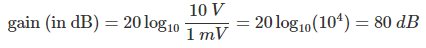A dB value is calculated differently when we want to express a power ratio: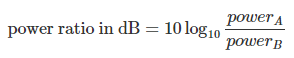## Expressing Absolute Quantities

We know from the preceding discussion that decibels always express a ratio, i.e., one value relative to another value. However, it is possible to express an absolute quantity in dB: all we have to do is choose a fixed reference value and modify “dB” so as to convey what that reference value is.

The unit dBW, for example, is a way of expressing an absolute quantity of power. The value in dBW is calculated by using 1W as the reference value: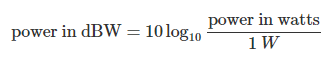Power in Watts Power Expressed as dBW 1 mW -30 dBW 1 W 0 dBW 1 kW 30 dBW 1 MW 60 dBW

## Review of Engineering Units

We’ve taken a fairly thorough look at the ways in which engineers discuss, write, and work with circuit quantities (such as voltage and current) and component values (such as capacitance and resistance).

In the next page, you’ll learn about a circuit-analysis technique that is used when resistors are connected together but are not in series or in parallel.